iOS Animation Contest 2021: Doubletapp Developer Experience
Consultation# iOS Animation Contest 2021: Doubletapp Developer Experience

In January 2021, Telegram announced the iOS Animation Contest 2021. The participant had to create animations in Telegram for iOS using the provided layouts. They had to optimize them on older devices and develop an editor with which to customize their functionality. Junior iOS developer Aleksey Muraveinik took part in the competition from Doubletapp. That's what came out of it.By:By:

Alexey Muraveynik## Contest in a nutshell

The organizers have provided photo and video files demonstrating animations that need to be implemented and embedded in the Telegram client on the iOS platform.

The code must be written in pure Swift; using third party UI frameworks like Flutter and React Native is prohibited.

### The task consists of several parts:

Implement animations for sending messages to chat

Messages should look like bubbles floating upImplement animation editor

In this editor, you can set the speed and movement of an animated object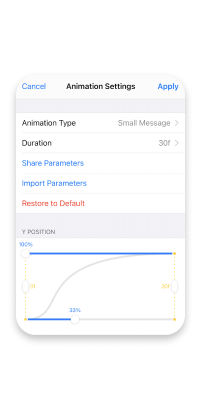Implement animated background

The background is a four-color gradient, the color centers of which are set at arbitrary points. Animation is obtained by moving color centersThe work is evaluated according to 3 criteria:

1. How similar are the implemented animations to those presented in the demo materials.
2. How smoothly animations play on modern devices.
3. How smoothly animations play on older devices (including the iPhone 6 and SE1).

The organizers give 16 days to complete the first task.

## Participation in the competition

It was interesting to participate in the contest from Telegram. The task at hand turned out to be difficult, requiring a special approach. I had to smash my head and look for solutions, but it was definitely worth it. the experience gained was not only fascinating but also useful.

On January 19, I was assigned to do some research on gradient rendering. It was immediately known that the standard method that I used in `` Edge '' and Practice probably won't work.

If you search Google for "swift gradient '', chances are you will find one of the following two ways:

The first method really justifies its position in the top the simplest gradient can be created in just 4 lines and get the following result

``````func createGradientLayer() {

}
``````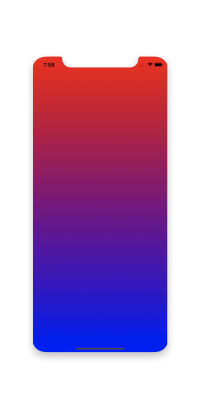``````func createGradientLayer() {

UIColor.red.cgColor,
UIColor.yellow.cgColor,
UIColor.blue.cgColor
]

gradientLayer.startPoint = CGPoint(x: 0, y: 0)
gradientLayer.endPoint = CGPoint(x: 1, y: 1)

}
``````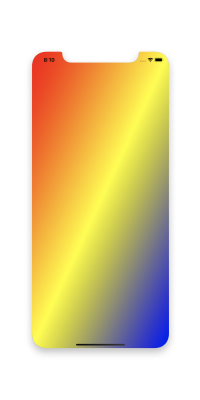Set transition points from one color to another

``````func createGradientLayer() {

UIColor.red.cgColor,
UIColor.purple.cgColor,
UIColor.blue.cgColor
]

gradientLayer.startPoint = CGPoint(x: 0, y: 0)
gradientLayer.endPoint = CGPoint(x: 1, y: 0)

}
``````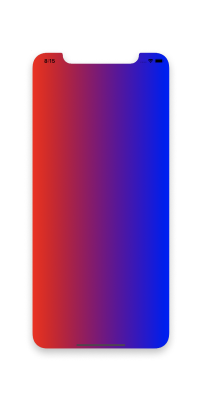For most, this functionality is sufficient, but not for us.

If you look closely at the background preview from the demo, you will notice that for each of the four colors a point is set, from which the color spreads in all directions. For each point, a radius is specified, within which the color does not change in any way in relation to the center color.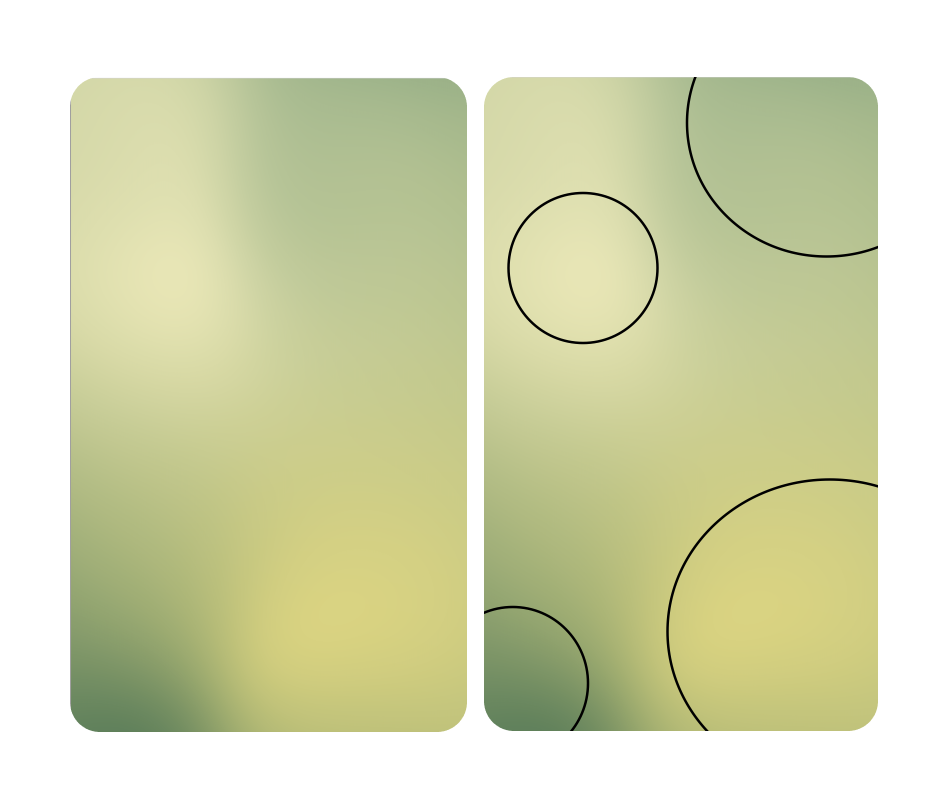This gives us a hint to look for a way to draw gradients not linearly, but in the form of circles.

Fortunately, there is a standard solution for implementing gradient rendering as circles. This method is more low-level and uses 2D drawing techniques.

``````import Foundation
import UIKit

override func draw(_ rect: CGRect) {
guard let currentContext = UIGraphicsGetCurrentContext(),
colors: [UIColor.red.cgColor, UIColor.blue.cgColor] as CFArray,
locations: [0.0, 1.0]
) else { return }

startCenter: CGPoint(x: self.bounds.width / 2, y: self.bounds.height / 2),
endCenter: CGPoint(x: self.bounds.width / 2, y: self.bounds.height / 2),
options: .drawsAfterEndLocation
)
}
}
``````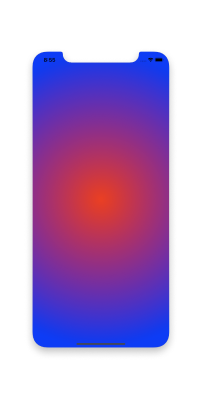Already somewhat remotely reminiscent of the circles that we need.

But the main problem with this approach is that these kind of gradients do not overlap each other, and instead of the expected overlap with the correct calculation of intermediate colors between the color centers, we get the following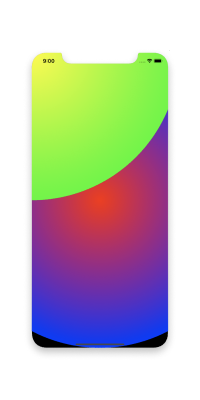Such a picture suggests that you need to come up with a solution that will calculate the color of each pixel on the screen.

### How color components are calculated in a gradient

Let's say we have 2 dots:

1. Red (R), RGB has the color (255, 0, 0).
2. Blue (B), RGB has color (0, 0, 255)

Let's select an arbitrary point for which we want to calculate the color. Let's call it P.

Then the calculation of RGB color components for P will be as follows:

• calculate the distance from R to B, let's call this distance RB;
• calculate the distance from R to P, let's call this distance RP;
• calculate the distance from B to P, let's call this distance BP;

Calculating color components for P:

• P.r = (RP / RB) * R.r + (BP / RB) * B.r;
• P.g = (RP / RB) * R.g + (BP / RB) * B.g;
• P.b = (RP / RB) * R.b + (BP / RB) * B.b.

This approach gives the expected result.

### What if the gradient is on a plane?

In our problem, 4 points of different colors are given, so we use the following principle for the calculation: `` Each specified color affects the color of any other point on the screen, if the distance from the center of the specified color to the point is less than some distance L.

I used CMYK (Cyan, Magenta, Yellow, Key) dots at the corners of the screen as the perfect four-color gradient to target.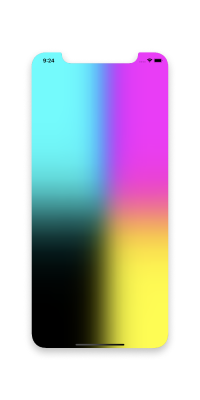Obviously, black (bottom left) should not affect turquoise (top left). It follows that L should be less than the screen height. But if we take the height as L, it turns out that turquoise will have an effect on the magenta, because the distance between them is screen width, i.e. less than L.

If we take the width as L, then there will be points on the screen that are more than L from all colors, and it is not known how to calculate the color for them.

Therefore, the decision comes to use the width of the screen as L, generate the gradient image itself in the shape of a square, and then stretch it to full screen.

Now for each point we calculate the influence factor of each of the colors on it.

This is done using the following formula:

• Let d be the distance from a point to a color.
• Let m be the maximum of {1 - (d / L)} and {0}. Here it turns out that if the distance from a point to a color is greater than L, then the influence factor of this color is zero.
• Let f = m ^ 4.

f is the desired factor. The degree in the formula is responsible for the smoothness / sharpness of color transitions. The higher the degree, the sharper the transition.

Now that we know the influence factor of each of the four colors on a point, we can calculate the color components using the following formula:

• Let f1, f2, f3, f4 be the factors influencing CMYK colors on a point
• Let s = f1 + f2 + f3 + f4
• Let sR = f1 * C.r + f2 * M.r + f3 * Y.r + f4 * K.r
• Let sG = f1 * C.g + f2 * M.g + f3 * Y.g + f4 * K.g
• Let sB = f1 * C.b + f2 * M.b + f3 * Y.b + f4 * K.b

Then the components of the point are:

• r = sR / s
• g = sG / s
• b = sB / s

Using this method, you can calculate the components for any point on the screen, and get the expected result.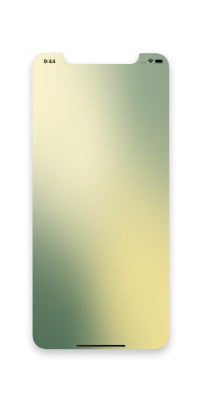Now that we are able to generate a gradient image with colors at arbitrary points, we can proceed with the animation itself.

Animation — these are changing pictures, that is, frames. The two standards for frame rate are considered to be 30 frames / s and 60 frames / s.
The demo animation shows that the color centers change for each frameThere are 8 points in total, the movement of colors to which occurs counterclockwise. For every two adjacent points, you need to generate 30 or 60 frames, in which the center of the color will be at one of the intermediate points.

And here we are faced with two problems.

### Frame generation optimization

At the moment, the algorithm for each frame calculates the number of dots equal to the screen width in a square. On iPhone 11, this number is 171396. And this number of color calculations for dots needs to be done in 1/60 of a second. Of course this is not possible. There are several steps to resolve this issue.

Caching

You can cache color influences for specified distances. Regardless of where the center of the color is located on the screen, the factor of its influence on a point located at a distance m from it will always be the same. Therefore, for the first time we consider the factors, and in all subsequent times just get the saved value.

Compression

You can reduce the width of the image by 10 times, and stretch the image itself to full screen. For example, on iPhone 11, the number of dots for which you need to calculate the color will be 1681. The gradient will still look as it should.

Buffering

If you prepare all frames at once before rendering, then the animation may delay at the beginning or memory will run out. If you prepare one frame at a time, then the gradient generator will not have time to form a new image during the period of time while the current one is being drawn, this will create a slowdown effect and kill the smoothness of the animation.

Solution: prepare 10 frames each and store them in the buffer. At the moment when the current 10 frames are being drawn, the generator draws new 10 frames and places them in the buffer. When the current frames are drawn, they are replaced with those in the buffer, and the generator draws 10 more and places them in the buffer

An example of the implementation of the mechanism for changing frames for animation
``````let timer = Timer.scheduledTimer(
withTimeInterval: 1 / Double(fps.rawValue), repeats: true) { [weak self] timer in

if i >= images.count {
images = buffer
buffer = []
self?.animationCreator.imagesRequired = true
i = 0
}

if i < images.count {
self?.imageView.image = images[i]

i += 1
} else if isFinished {
timer.invalidate()
self?.isAnimating = false
return
}
}
``````

### Nonlinear movement of color centers

As you can see on the demo materials points movement graph curve, that is, the points move nonlinearly. The competition did not specify any function that could describe such a movement, so it was decided to set the function in discrete form.Horizontal axis timeline, we divide it into 60 parts (corresponds to 60 frames per second).

Vertical axis the scale of movement, we divide it into 27 parts (conventionally).

Then, in order to find the center of the color moving from point A to point B, for each of 60 frames do the following:

• Let dx = (B.x - A.x) / 27
• Let dy = (B.y - A.y) / 27

Define the animationCurve display:

Mapping the frame number to the factor of the point movement unit

And the center of the color for each frame is defined as

x =  A.x + dx * animationCurve [frameNumber]

y = A.y + dy * animationCurve [frameNumber]

### Summarizing

1. Caching the influencing factors of the colors.
2. Generate a square image of size {(screen width) / 10}.
2. Animation
1. Storing 10 frames into the buffer while the current 10 frames are being drawn
2. The nonlinearity of moving points is described using a discrete function

## Final result## Conclusion

It was difficult, but interesting.

The code for the final version of this project (and not only this one 😉) can be found in our GitHub repositories

Discuss article on social media

Similar news

### step 1: choose service

mobile development

web development

machine learning

design

audit

technical requirenment

consultation

animation